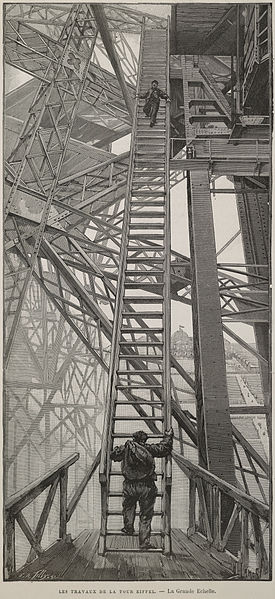Probabilistic Steps (Random Walk) Again!

Probability Level 5A man is standing on an infinite ladder. In one move, he can either go up (with probability $0.4$), down (with probability $0.5$) or stay where he is (with probability $0.1$).

Find the probability that after $11$ moves he is one step away from his initial position.

Inspired by this problem.

×